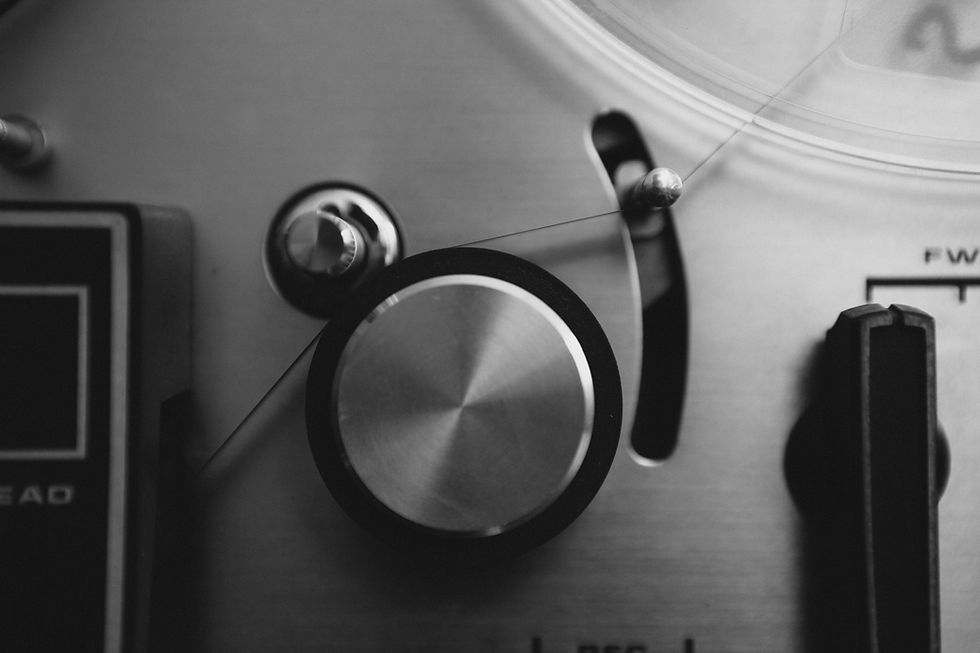top of page
Search
• Alibek Jakupov

# Information Theory: Step 7 Add noise

Updated: Nov 19, 2021In this article we are going to create a simple noise generator to set up our future experiment.

As we have seen before, the main goal of this series of articles is to create a solid and error-prone communication system.

Error control are the techniques that allow us guarantee our users a certain level of quality even the communication channel is unreliable.

We have seen error detection and error correction techniques based on Hamming code. We have also implemented error detection based on Hamming distance and syndrome calculation.

So, if you remember, we have seen that theses techniques are based on the principle of adding so-called parity bits that in fact allow us to implement error detection.

The final transformation looks like:

(i1, i2, i3, i4) -> (i1, i2, i3, i4, r1, r2, r3)

where i corresponds to the data bit (our initial message), and r is a parity bit.

Formula for calculating parity bits:

first parity bit = i1 XOR i2 XOR i3 (where i is a symbol from original message)
second parity bit = i2 XOR i3 XOR i4
third parity bit = i2 XOR i3 XOR i4

We then calculate the error syndrome which will help us detect erroneous symbol.

In this series we developed (7,4) Hamming code, in other words, to code correctly a message of 4 symbols (k) we need 3 parity bits, thus making a 7 (n) symbols message.

Important: with this configuration the algorithm is only able to correct a single error. However, an error is corrected in the encoded message, thus even if there was en error in parity bits we will be able to detect and correct it.

This doesn't mean that only a single error in the whole message is detected and corrected. It actually corrects a single error per block. Thus, if you have a message of 1024 bits, it means that your encoded message will be divided into 256 blocks with 3 parity bits added to each block, and the system will be able to correct up to 256 errors.Not surprisingly, we want to simulate these conditions but how do we find a transmission channel that generates noise in our date. For the experimentation purpose we created a simple script that adds error in each message block at a random position.

```import math
from random import randint

def noize(sequence):
number_of_blocks = len(sequence)/7
temp = list(sequence)
for i in range (0, number_of_blocks):
end  = (i+1)*7 - 1
start = (i+1)*7 -7
index = randint(start,end)
print index
if (temp[index]=='0'):
temp[index]='1'
elif (temp[index]=='1'):
temp[index]='0'
sequence = "".join(temp)
print sequence
def swap(digit):
if digit == '0':
digit = '1'
if digit == '1':
digit = '0'

x  = raw_input("Enter your code: ")
noize(x)```

In the next (an final) article we are going to implement error correction based on Hamming algorithm.

P.S. Of course, link to the github. Enjoy